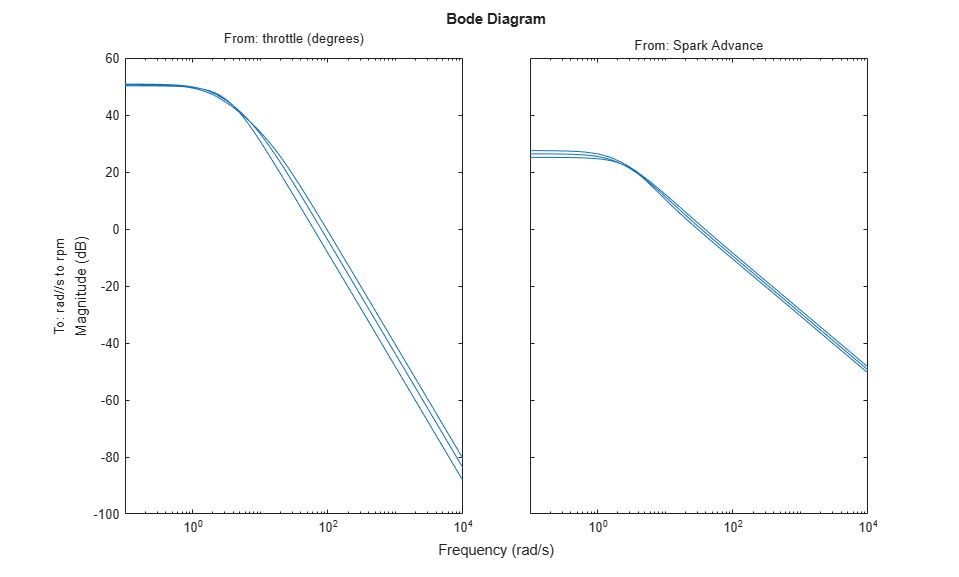# Linearize Engine Speed Model

This example shows how to linearize an engine speed model for multiple output conditions.

### Engine Speed Model

Open the model.

```mdl = 'scdspeed'; open_system(mdl) ```For this example, you find linear models from the spark advance and throttle angle inputs to the output engine speed. You do so for three speed conditions: 2000, 3000, and 4000 rpm.

### Find Operating Points

Create an array of three operating point specifications.

```opspec = operspec(mdl,[3 1]); ```

Since the Simulink® model does not have any root-level inports, `opspec` does not contain any output specifications. You can add output specifications for a given signal in your model using the `addoutputspec` function.

Add an output specification to the output of the rad/s to rpm block.

```opspec = addoutputspec(opspec,'scdspeed/rad//s to rpm',1); ```

For each specification, indicate that the output value is known and specify the output value. Set the known speed values to 2000, 3000, and 4000 rpm.

```opspec(1).Outputs.Known = 1; opspec(1).Outputs.y = 2000; opspec(2).Outputs.Known = 1; opspec(2).Outputs.y = 3000; opspec(3).Outputs.Known = 1; opspec(3).Outputs.y = 4000; ```

View the specifications object for the third operating condition.

```opspec(3) ```
```ans = Operating point specification for the Model scdspeed. (Time-Varying Components Evaluated at time t=0) States: ---------- x Known SteadyState Min Max dxMin dxMax ___________ ___________ ___________ ___________ ___________ ___________ ___________ (1.) scdspeed/Throttle & Manifold/Intake Manifold/p0 = 0.543 bar 0.543 false true -Inf Inf -Inf Inf (2.) scdspeed/Vehicle Dynamics/w = T//J w0 = 209 rad//s 209.48 false true -Inf Inf -Inf Inf Inputs: ---------- u Known Min Max _____ _____ _____ _____ (1.) scdspeed/Throttle perturbation 0 false -Inf Inf Outputs: ---------- y Known Min Max _____ _____ _____ _____ (1.) scdspeed/rad//s to rpm 4000 true -Inf Inf ```

Search for operating points that meet these specifications using the `findop` function.

```opt = findopOptions('DisplayReport','off'); op = findop(mdl,opspec,opt); ```

View the resulting operating point for the third operating condition.

```op(3) ```
```ans = Operating point for the Model scdspeed. (Time-Varying Components Evaluated at time t=0) States: ---------- x _______ (1.) scdspeed/Throttle & Manifold/Intake Manifold/p0 = 0.543 bar 0.4731 (2.) scdspeed/Vehicle Dynamics/w = T//J w0 = 209 rad//s 418.879 Inputs: ---------- u ______ (1.) scdspeed/Throttle perturbation 5.8292 ```

### Linearize Model

To linearize the model, first specify the linearization input points at the outputs of the throttle and Spark Advance blocks.

```io(1) = linio('scdspeed/throttle (degrees)',1,'input'); io(2) = linio('scdspeed/Spark Advance',1,'input'); ```

Next, specify the linearization output point at the output of the rad/s to rpm block.

```io(3) = linio('scdspeed/rad//s to rpm',1,'output'); ```

Linearize the model for each of the operating conditions.

```sys = linearize(mdl,op,io); ```

Plot the Bode magnitude response for the resulting linear models.

```bodemag(sys) ```Close the model.

```bdclose(mdl) ```